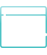Domain Registration in Pakistan | Secure Your Online Presence

# DOMAIN REGISTRATION IN PAKISTAN## Best Hosting

### WHY CHOOSE US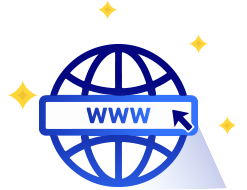#### One place for all TLDs registration

HostingWalay offer multiple types of TLDs, ccTLDs, sTLDs and gTLDs for registration and transfer purpose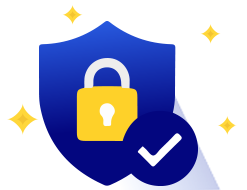#### Instant activation with Registrar Lock

Activate your domain name instantly with registrar lock to safe it from being transferred to anyone#### Free two personalized Email Accounts

Activate any TLD with two personalized Email Addresses including with spam & virus free protection

## HostingWalay

### Lowest and Affordable Domain Names

Domain (TLD) Registration Price
.pw Rs. 324.00
.in.net Rs. 400.00
.fun Rs. 425.00
.space Rs. 475.00
.podhale.pl Rs. 545.00
.wolomin.pl Rs. 545.00
.podlasie.pl Rs. 545.00
.polkowice.pl Rs. 545.00
.pomorskie.pl Rs. 545.00
.wroclaw.pl Rs. 545.00
.pomorze.pl Rs. 545.00
.beskidy.pl Rs. 545.00
.malbork.pl Rs. 545.00
.malopolska.pl Rs. 545.00
.bialowieza.pl Rs. 545.00
.bialystok.pl Rs. 545.00
.mazowsze.pl Rs. 545.00
.zachpomor.pl Rs. 545.00
.bielawa.pl Rs. 545.00
.mazury.pl Rs. 545.00
.zagan.pl Rs. 545.00
.zarow.pl Rs. 545.00
.zgora.pl Rs. 545.00
.elblag.pl Rs. 545.00
.prochowice.pl Rs. 545.00
.zgorzelec.pl Rs. 545.00
.elk.pl Rs. 545.00
.pruszkow.pl Rs. 545.00
.przeworsk.pl Rs. 545.00
.pulawy.pl Rs. 545.00
.boleslawiec.pl Rs. 545.00
.mielec.pl Rs. 545.00
.mielno.pl Rs. 545.00
.rawa-maz.pl Rs. 545.00
.bytom.pl Rs. 545.00
.mragowo.pl Rs. 545.00
.naklo.pl Rs. 545.00
.rybnik.pl Rs. 545.00
.rzeszow.pl Rs. 545.00
.sanok.pl Rs. 545.00
.cieszyn.pl Rs. 545.00
.sejny.pl Rs. 545.00
.glogow.pl Rs. 545.00
.gniezno.pl Rs. 545.00
.gorlice.pl Rs. 545.00
.grajewo.pl Rs. 545.00
.skoczow.pl Rs. 545.00
.slupsk.pl Rs. 545.00
.sosnowiec.pl Rs. 545.00
.stalowa-wola.pl Rs. 545.00
.starachowice.pl Rs. 545.00
.stargard.pl Rs. 545.00
.nowaruda.pl Rs. 545.00
.nysa.pl Rs. 545.00
.suwalki.pl Rs. 545.00
.ilawa.pl Rs. 545.00
.olawa.pl Rs. 545.00
.swidnica.pl Rs. 545.00
.olecko.pl Rs. 545.00
.swiebodzin.pl Rs. 545.00
.olkusz.pl Rs. 545.00
.swinoujscie.pl Rs. 545.00
.olsztyn.pl Rs. 545.00
.szczecin.pl Rs. 545.00
.szczytno.pl Rs. 545.00
.opoczno.pl Rs. 545.00
.opole.pl Rs. 545.00
.tarnobrzeg.pl Rs. 545.00
.tgory.pl Rs. 545.00
.jaworzno.pl Rs. 545.00
.jelenia-gora.pl Rs. 545.00
.jgora.pl Rs. 545.00
.kalisz.pl Rs. 545.00
.karpacz.pl Rs. 545.00
.kartuzy.pl Rs. 545.00
.kaszuby.pl Rs. 545.00
.katowice.pl Rs. 545.00
.kazimierz-dolny.pl Rs. 545.00
.turek.pl Rs. 545.00
.kepno.pl Rs. 545.00
.ketrzyn.pl Rs. 545.00
.tychy.pl Rs. 545.00
.klodzko.pl Rs. 545.00
.kobierzyce.pl Rs. 545.00
.kolobrzeg.pl Rs. 545.00
.konin.pl Rs. 545.00
.konskowola.pl Rs. 545.00
.ustka.pl Rs. 545.00
.kutno.pl Rs. 545.00
.lapy.pl Rs. 545.00
.lebork.pl Rs. 545.00
.ostroda.pl Rs. 545.00
.ostroleka.pl Rs. 545.00
.legnica.pl Rs. 545.00
.ostrowiec.pl Rs. 545.00
.lezajsk.pl Rs. 545.00
.ostrowwlkp.pl Rs. 545.00
.czest.pl Rs. 545.00
.limanowa.pl Rs. 545.00
.walbrzych.pl Rs. 545.00
.warmia.pl Rs. 545.00
.warszawa.pl Rs. 545.00
.waw.pl Rs. 545.00
.lomza.pl Rs. 545.00
.lowicz.pl Rs. 545.00
.wegrow.pl Rs. 545.00
.pila.pl Rs. 545.00
.wielun.pl Rs. 545.00
.pisz.pl Rs. 545.00
.lubin.pl Rs. 545.00
.dlugoleka.pl Rs. 545.00
.lukow.pl Rs. 545.00
.wlocl.pl Rs. 545.00
.wloclawek.pl Rs. 545.00
.wodzislaw.pl Rs. 545.00
.shop Rs. 557.00
.mom Rs. 627.00
.homes Rs. 627.00
.lol Rs. 627.00
.pics Rs. 627.00
.world Rs. 671.00
.life Rs. 675.00
.today Rs. 677.00
.mail.pl Rs. 764.00
.powiat.pl Rs. 764.00
.priv.pl Rs. 764.00
.edu.pl Rs. 764.00
.biz.pl Rs. 764.00
.media.pl Rs. 764.00
.miasta.pl Rs. 764.00
.mil.pl Rs. 764.00
.realestate.pl Rs. 764.00
.rel.pl Rs. 764.00
.gmina.pl Rs. 764.00
.sex.pl Rs. 764.00
.shop.pl Rs. 764.00
.sklep.pl Rs. 764.00
.gsm.pl Rs. 764.00
.net.pl Rs. 764.00
.sos.pl Rs. 764.00
.nieruchomosci.pl Rs. 764.00
.nom.pl Rs. 764.00
.szkola.pl Rs. 764.00
.targi.pl Rs. 764.00
.info.pl Rs. 764.00
.tm.pl Rs. 764.00
.com.pl Rs. 764.00
.tourism.pl Rs. 764.00
.travel.pl Rs. 764.00
.turystyka.pl Rs. 764.00
.org.pl Rs. 764.00
.pc.pl Rs. 764.00
.xyz Rs. 798.00
.pl Rs. 884.00
.pro Rs. 920.00
.msk.ru Rs. 924.00
.guru Rs. 945.00

### Domain Freebies

#### Get over Rs 6000 worth of Free Services with every Domain name you order with HostingWalay!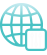#### DNS Management

Get free DNS service to manage your records on our globally DNS infrastructure.#### Mail Forwarding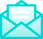#### Email Account

Enjoy 2GB free email account with every domain you order without hosting account.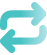#### Unauthorized Transfer

Protect your domain from unauthorized transfer, or "hijack" with our free Domain Theft Protection.#### Domain Forwarding